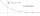# Geometric progression + power - math problems

#### Number of problems found: 10

• Three members GPThe sum of three numbers in GP (geometric progression) is 21 and the sum of their squares is 189. Find the numbers.
• What is 10What is the 5th term, if the 8th term is 80 and common ratio r =1/2?
• Rate or interestAt what rate percent will Rs.2000 amount to Rs.2315.25 in 3 years at compound interest?
• What are 2What are the two more terms of the GP a, ax, ax2, ax3, __, __?
• If the 3If the 6th term of a GP is 4 and the 10th is 4/81, find common ratio r.
• SaleIf the product twice price cut by 25%, what percentage was price cut in total?
• Sum of GP membersDetermine the sum of the GP 30, 6, 1.2, to 5 terms. What is the sum of all terms (to infinity)?
• Future valueSuppose you invested \$1000 per quarter over a 15 years period. If money earns an anual rate of 6.5% compounded quarterly, how much would be available at the end of the time period? How much is the interest earn?
• The half lifeThe half-life of a radioactive isotope is the time it takes for a quantity of the isotope to be reduced to half its initial mass. Starting with 145 grams of a radioactive isotope, how much will be left after 3 half-lives?
• Present valueA bank loans a family \$90,000 at 4.5% annual interest rate to purchase a house. The family agrees to pay the loan off by making monthly payments over a 15 year period. How much should the monthly payment be in order to pay off the debt in 15 years?

We apologize, but in this category are not a lot of examples.
Do you have an interesting mathematical word problem that you can't solve it? Submit a math problem, and we can try to solve it.

We will send a solution to your e-mail address. Solved examples are also published here. Please enter the e-mail correctly and check whether you don't have a full mailbox.

Please do not submit problems from current active competitions such as Mathematical Olympiad, correspondence seminars etc...

Geometric progression - math word problems. Power - math word problems.